# Orthogonal

## Riemannian General Relativity [Extra]

Orthogonal

This page is aimed at readers who are already reasonably familiar with the terminology and results of general relativity, as applied to our own universe. If you’re interested in quickly learning the basics of that subject, try the Foundations essays on special and general relativity. If you just want a summary of the relativistic gravitational phenomena that are different in the Riemannian universe, try the main page on this topic.

### The Riemannian Schwarzschild Solution

Einstein’s equation linking the curvature of space-time to the matter it contains is:

G + Λ g = 8 π T

where:

We are following common practice in general relativity and using units where not only are time and space measured in the same units as each other, mass is as well, and the units are chosen so that the gravitational constant (which, rather confusingly, is often given the symbol G that is also used for the Einstein tensor) becomes equal to 1.

There is no reason that this equation needs to change to be applied in the Riemannian universe. The strength of gravity in relation to other forces might or might not be different in the Riemannian universe, but since we’ve already absorbed the gravitational constant into the choice of units — whatever its value — there’s nothing more to be done about that. The most important feature of Einstein’s equation is that the divergence of Einstein’s tensor is necessarily zero, and the same is true of any constant times the metric. This allows these quantities to be sensibly equated to a constant multiple of the stress-energy tensor, whose divergence must be zero in order for local conservation of energy and momentum to hold. All of this will be equally true, and equally appropriate, in the Riemannian universe.

So the only real difference is that we will require solutions of Einstein’s equation in which the metric g has a signature of (++++): that is, solutions for which all dimensions are spacelike.

Of course there is one possible change that could be made without disturbing the divergence-free property of the left-hand side of the equation: we could require the gravitational constant to be negative, and so even when its magnitude was set to 1 by the choice of units, the right-hand side of the equation would have a minus sign. But as we’ll see, if we want gravity in the Riemannian universe to behave essentially like Newtonian gravity when objects are moving slowly, keeping a positive gravitational constant turns out to be the correct choice.

In many contexts in our own universe the cosmological constant Λ can be taken to be zero, but we’ll keep things as general as possible by including it in our calculations, only setting it to zero to obtain some specific results. So we’ll start our exploration of gravity by presenting the “Schwarzschild-Kottler” metric, which is a generalisation of the Schwarzschild metric to include a cosmological constant.

 The Schwarzschild-Kottler Metric r, θ, φ are polar coordinates centred on body of mass M g = (1+2M/r–Λr2/3) dt2 + 1/(1+2M/r–Λr2/3) dr2 + r2 [dθ2 + (sin θ)2 dφ2] (Riemannian) g = –(1–2M/r–Λr2/3) dt2 + 1/(1–2M/r–Λr2/3) dr2 + r2 [dθ2 + (sin θ)2 dφ2] (Lorentzian)

This metric describes the geometry in the vacuum outside a spherically symmetrical body with mass M. For now we will remain uncommitted to any specific value, or even sign, of Λ in the Riemannian universe, but we will assume that |Λ| is small enough that 1+2M/r–Λr2/3 remains positive over the region of interest.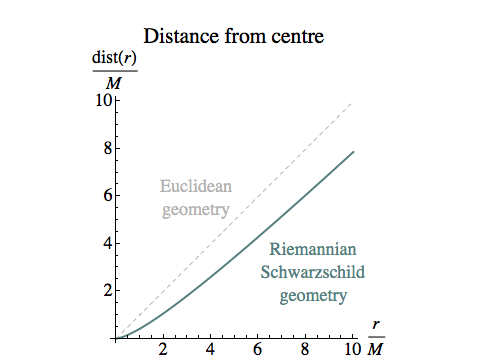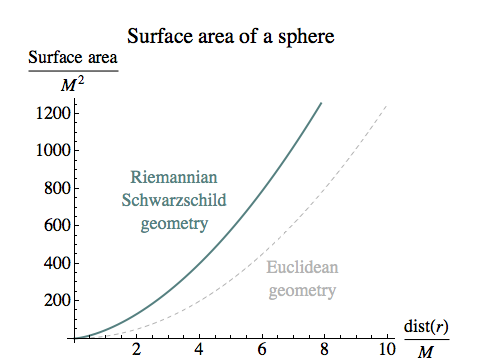One striking difference between the Lorentzian and Riemannian metrics is that in the Riemannian case, all radial distances measured along paths of constant t, θ and φ are finite. For Λ=0 we can even get an explicit formula for the distance from the centre of the coordinates (assuming the solution holds all the way down to r=0):

dist(r) = √[r (r + 2 M)] – 2 M arcsinh(√[r / (2 M)])

The area of a surface of constant r in this metric is simply 4 π r2, but the value of dist(r) is always less than r itself, so these spheres have a greater surface area for their radius than they would have in Euclidean geometry. This is a characteristic of a space with negative curvature.

In contrast to this, radial distances in the Lorentzian Schwarzschild metric aren’t even spacelike unless r > 2 M, and then they are always greater than the difference in the r coordinate.

#### Constants of Motion and the Effective Potential

We’d like to understand the motion of a small “test particle” in this metric: an orbiting (or infalling) body that is small enough to have a negligible effect on the geometry itself. The general method we follow will be close to Misner, Thorne and Wheeler in their treatment of a body moving in the ordinary Schwarzschild geometry.

Our test particle’s world line will be a geodesic, and we will use the symbol u to denote its 4-velocity: the unit vector tangent to its world line. The components of the vector u in our coordinate system are simply the rate of change with proper time τ, along the geodesic, of the coordinates:

ut = ∂τ t
ur = ∂τ r
uφ = ∂τ φ
uθ = ∂τ θ

Because the geometry is spherically symmetrical, we can always choose coordinates so that the world line of the test particle lies in the “equatorial plane” at θ=π/2, and uθ = ∂τ θ = 0.

Since the metric is independent of the coordinates t and φ, the coordinate vector fields ∂t and ∂φ are Killing vector fields: fields that describe ways of “sliding” a figure through the geometry while preserving its shape and size, in the same way that we can imagine, say, adding 5 degrees to the longitude of every point on an island without distorting its geography. (For an informal discussion of Killing vector fields, try this page; though it starts with a quote from my novel Incandescence, it doesn’t actually require any familiarity with that book.)

A consequence of ∂t and ∂φ being Killing vector fields is that the dot product of the tangent to any geodesic with these fields will remain constant along the length of the geodesic. So for the entire world line of our test particle, we will have:

u · ∂t = ut = E
u · ∂φ = uφ = L

where we’ve introduced E and L as names for the two constants. The low-index components ut and uφ are components of the dual vector corresponding to u. The fact that u is a unit vector, and its dual vector also has a length of 1, is expressed in terms of the dual components as:

gij ui uj = 1

where we’re using the Einstein Summation Convention. Since the metric is diagonal, the upper-index metric components gij are just the reciprocals of the lower-index components we gave in the definition of the Schwarzschild-Kotter metric, so we have:

gtt ut2 + grr ur2 + gφφ uφ2 = 1
E2 / (1+2M/r–Λr2/3) + (1+2M/r–Λr2/3) ur2 + L2 / r2 = 1

If we express ur in terms of ur = ∂τ r, we have:

ur = grr ur = (∂τ r) / (1+2M/r–Λr2/3)

and hence:

[E2 + (∂τ r)2] / (1+2M/r–Λr2/3) + L2 / r2 = 1

or

 (∂τ r)2 = (1 – L2 / r2) (1+2M/r–Λr2/3) – E2 (1)

So we have an equation linking the rate of change of r with the time τ measured by the test particle, and the particle’s r coordinate. By differentiating this with respect to τ (and then dividing both sides by 2 ∂τ r) we obtain the second derivative:

 ∂τ,τ r = L2 (r+3M)/r4 – M/r2 – Λ r/3 (2)

The first term represents centrifugal acceleration, a roughly inverse-cube repulsion for a given amount of angular momentum L. In the Lorentzian version the numerator here is r–3M, so the term changes sign at r=3M, which is why there can be no orbits around a black hole closer than that. But here, centrifugal force stays centrifugal everywhere, and is always stronger than the Lorentzian and Newtonian versions.

The second term is just inverse-square gravitational attraction, so we can see that we have the sign of M correct if we want gravity to have the usual Newtonian limit.

And the third term is a cosmological-constant-based attraction or repulsion, which can either strengthen or weaken gravity at large scales according to the sign of Λ.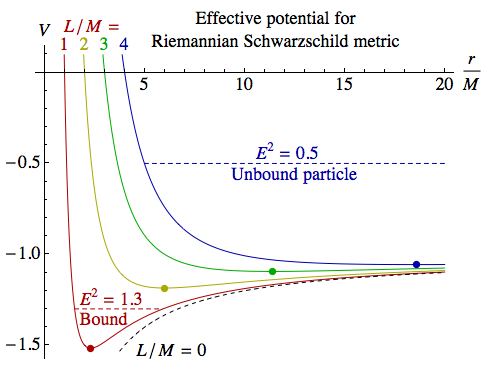The plot on the right shows the effective potential for the test particle’s radial motion, in the case Λ=0, for a range of values for L. The function:

V(r) = (L2 / r2 – 1) (1 + 2M/r)

determines the radial motion through equation (1), which becomes:

(∂τ r)2 + V(r) = –E2

For every non-zero value of L the effective potential V has a minimum, where V < –1. So any particle with E > 1 and L≠0 will be in a stable, bound orbit, moving back and forth between the values of r for which ∂τ r = 0, or V(r) = –E2. The dashed red line in the diagram shows an example for E2 = 1.3, L = M.

If E < 1, the particle will not be bound and will escape to infinity, with its closest approach where V(r) = –E2. The dashed blue line in the diagram shows an example for E2 = 0.5, L = 4M. E is the total energy of the particle divided by its rest mass, as measured at infinity in a reference frame in which the central mass is at rest. In Lorentzian physics a particle is bound when its energy is less than its rest mass, corresponding to E < 1, but in Riemannian physics the pattern is reversed: a bound particle has a greater energy than a free one.

#### Circular Orbits

Suppose we have a test particle in a circular orbit around the central mass. Then r=R is a constant and the first and second derivatives of r become zero, giving us two equations for L2 and E2 in terms of the orbital radius R. If we solve these equations and insert the results into the 4-velocity u, we can then determine the ordinary velocity of the test particle, as judged by a motionless observer at the same distance from the central body. The result is: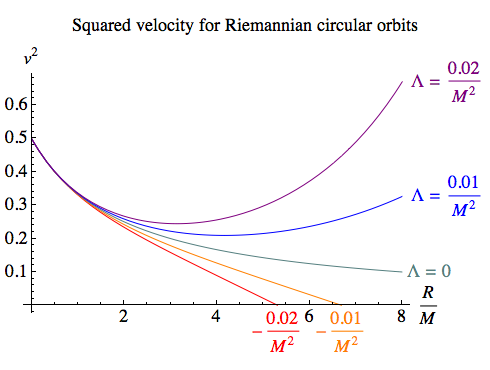v2 = (3 M + Λ R3) / (3 R + 6 M – Λ R3)

For the case of Λ=0 this becomes:

v2 = M / (R + 2 M)

For large R and Λ=0 this gives the Newtonian limit, where the kinetic energy is half the magnitude of the negative gravitational potential energy.

In Newtonian physics, as the orbiting body gets closer to the central mass its velocity increases without bound. The Riemannian orbits, though, all have v2 approaching 1/2 as R gets smaller. Because centrifugal force is stronger (taking on an inverse-fourth-power character for small r), 1/√2 is the fastest that any object can be travelling in order to be bound in a circular orbit (at least close up).

If there is a cosmological constant, there are modifications for sufficiently large orbits. If Λ is positive, gravity becomes stronger on large scales, and after falling to a minimum v2 starts to rise again, without bound. If Λ is negative, the cosmological term adds a repulsion that eventually makes it impossible to gravitationally bind an orbiting body, no matter how slowly it is moving.

#### Radial Motion and Escape Velocity

Suppose our test particle is moving directly towards or away from the central body. Then L = 0, and we can determine the value of the other constant of motion, E2, in terms of the velocity it has at some particular value for r (judged by a motionless observer at the same distance).

E2 = (1 + 2M / r – Λ r2 / 3) / (1 + v2)

which for Λ=0 becomes:

E2 = (1 + 2M / r) / (1 + v2)

In the Λ=0 case, E2 for a particle just able to escape to infinity is the value it takes when v→0 as r→∞, which is 1. So a particle’s escape velocity is given by:

vesc2 = 2M / r

This matches the Newtonian form of the escape velocity — and makes it clear that there is no distance from which escape is impossible.

In the more general case, with Λ≠0, “escape velocity” isn’t really a meaningful notion, because the cosmological term will either be repelling or attracting the particle at large distances. However, regardless of what’s going on at cosmological scales, it remains true that the central mass itself will never be impossible to escape. There are no Riemannian black holes.

#### Gravitational Blueshift and Time Dilation

If a beam of light very far from the central mass is approaching it with a speed v, then (assuming Λ=0, so we have well-defined behaviour at r→∞), it will certainly be travelling faster when it reaches a distance r, with:

1 / (1 + v2) = (1 + 2M / r) / (1 + v(r)2)
v(r)2 = (1 + 2M / r) v2 + 2M / r

Faster light will have a shorter wavelength, which in the language of Orthogonal is described as blue-shifted. But unlike the Lorentzian case, this shorter wavelength will be accompanied by a longer period τ, and each cycle of the light will be measured as taking a longer time, close to the massive body. Specifically:

τ(r) = τ √(1 + 2M / r)

It follows that someone who takes a detour in space that brings them close to a massive body will experience more time passing overall than someone who avoids the detour. As with other time dilation effects due to motion in flat four-space, gravitational time dilation in the Riemannian universe means more time for the traveller, not less.

#### Gravitational Scattering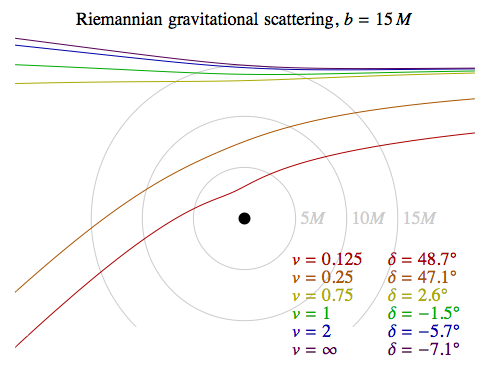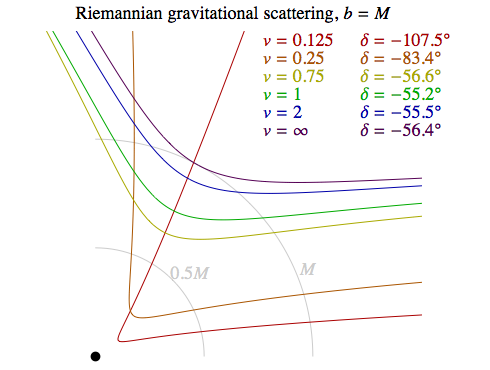In Newtonian gravitational physics, a test particle that travels towards a massive body from far away — and is offset sufficiently to avoid a collision — will follow a hyperbolic trajectory that curves towards the central mass. When the particle escapes to infinity again, the direction in which it’s travelling will have changed. We say the particle has been scattered by a certain angle, which we’ll call δ.

In Lorentzian gravitation similar trajectories are the norm, but in the extreme case of a black hole the particle can swing around the hole several times before escaping or being captured.

In Riemannian gravitation there’s no such thing as a black hole, but when the encounter involves massive, compact objects or fast-moving particles there is deflection away from the body, rather than towards it.

The plots on the right show trajectories for test particles with a variety of initial velocities, v, and two values for the impact parameter, b, which is defined as the sideways displacement of the trajectory from a head-on collision (as measured when the particle is at infinity). The red and orange curves in the top diagram — for reasonably slow-moving particles that don’t get too close to the massive body — resemble Newtonian hyperbolas, and the yellow curve on the same diagram only deviates slightly from its original direction, but all the other trajectories are deflected away from the central mass.

What flings these particles away is a non-Newtonian centrifugal force. As we saw with equation (2), for small values of r the centrifugal acceleration for a given angular momentum L will be roughly proportional to the inverse-fourth-power of r, as opposed to the inverse-cube in Newtonian physics.

### Cosmology

#### Curvature and Entropy

Starting with Einstein’s equation, we can derive a useful expression for the Ricci tensor in terms of the stress-energy tensor. The Einstein tensor G is defined in terms of the Ricci tensor R and the metric g by:

G = R – (1/2) Rcc g

where we’re using the Einstein Summation Convention to take the trace of the Ricci tensor. If we write Einstein’s equation in component form, we have:

Gab + Λ gab = 8 π Tab
Rab – (1/2) Rcc gab + Λ gab = 8 π Tab

The trace of the stress-energy tensor can then be found as:

8 π Tee = Ree – (1/2) Rcc gee + Λ gee

The trace gee for a Riemannian metric is just the dimension of the space, 4. So we have:

8 π Tee = Ree – 2 Rcc + 4 Λ = –Ree + 4 Λ

[Of course for a 4-dimensional Lorentzian space, gee = 2, using the (– + + +) signature convention. So the equation above and those that follow do not apply in a Lorentzian universe.]

Making use of this, we can write:

8 π (Tab – (1/2) Tee gab)
= 8 π Tab + [(1/2) Ree – 2 Λ] gab

Then inserting the value of 8 π Tab from Einstein’s equation, we have:

8 π (Tab – (1/2) Tee gab)
= Rab – (1/2) Rcc gab + Λ gab + [(1/2) Ree – 2 Λ] gab
= Rab – Λ gab

This gives us the Ricci tensor in terms of the stress-energy tensor and the metric:

 Rab = 8 π (Tab – (1/2) Tee gab) + Λ gab (3)

Rearranging our earlier result connecting the traces of the Ricci and stress-energy tensors:

 Rcc = –8 π Tcc + 4 Λ (4)

As well as the trace of the Ricci tensor, we’ll be interested in its individual diagonal elements. If we work in locally orthonormal coordinates so that gaa = 1 for each a, we have (no summation on the pairs of repeated low indices):

 Raa = 8 π (Taa – (1/2) Tcc) + Λ (5)

What Raa tells us is how a bundle of initially parallel geodesics aligned in the a direction will come together or spread apart, in the sense that the volume they enclose (when sliced by a hypersurface orthogonal to the a direction) has a second rate of change that is positive, negative or zero. More precisely:

(∂aa V ) / V = –Raa

Now, roughly speaking we can classify matter in the Riemannian universe into two broad categories. In a low-entropy state where most matter is moving in more or less the same direction (a solid, a low-negative-temperature gas, or a system of gravitationally bound objects), if we align our time coordinate t with the world lines of this matter, the stress-energy tensor will have a large value for Ttt, and negligible values for the other components. An example of this would be a dust cloud of density ρ, with negligible pressure:

T = ρ uu

which in an orthonormal reference frame where the cloud is motionless has Ttt = ρ and all other components zero. We then have:

Tcc = ρ
Rtt = 4 π ρ + Λ
Rxx = Ryy = Rzz = –4 π ρ + Λ
Rcc = –8 π ρ + 4 Λ

If Λ=0, then Rtt > 0, so the volume enclosed by a bundle of geodesics lined up in the t direction will shrink. In other words, gravity will be attractive for clusters of test particles starting out at rest with respect to the dust cloud, bringing them together. Rxx etc. will be negative, though, so clusters of test particles moving through the dust cloud with an infinite velocity will become spread out. The trace of the Ricci tensor, also known as the scalar curvature, will be negative.

A sufficiently positive value for Λ would make clusters of test particles contract whatever their direction, and yield a positive scalar curvature, while a sufficiently negative value could reverse all those results.

What if matter is in a high-entropy state, equally likely to have its world line pointing in any direction in four-space? In such a state, we have:

Ttt = Txx = Tyy = Tzz = ρ

High-entropy matter in the Riemannian universe acts just like a cosmological constant: its stress-energy tensor is simply a multiple of the metric. Combining the effects of ρ and Λ we have:

Tcc = 4 ρ
Rtt = Rxx = Ryy = Rzz = –8 π ρ + Λ
Rcc = –32 π ρ + 4 Λ

If Λ is zero, then the scalar curvature will be negative, as will all the individual Ricci curvatures Raa, so bundles of geodesics aligned in any direction will move apart. If ρ is constant throughout four-space, that would make the Riemannian universe a four-dimensional hyperbolic manifold.

A sufficiently positive value for Λ could overcome the effect of ρ and yield positive curvature, while a negative value for Λ would act like an increase in ρ and exacerbate the negative curvature.

#### Simple Models

##### Big Bang Models

It appears to be possible to have a Big Bang singularity in Riemannian cosmology. However, unlike the case in Lorentzian physics (where a singularity seems to be unavoidable), it only happens if a certain kind of symmetry is imposed on the matter in the universe.

If we assume a perfectly homogeneous and isotropic spatial universe in the form of a 3-sphere, Euclidean space or hyperbolic space with a length scale a(t) that changes over time (as developed in Misner, Thorne and Wheeler for the Lorentzian case), Einstein’s equation becomes a differential equation for a(t):

 Dynamics of Homogeneous, Isotropic Universe k = 1 for a 3-spherek = 0 for Euclidean spacek = –1 for hyperbolic space 3 a'(t)2 / a(t)2 – 3 k / a(t)2 + Λ = 8 π ρ(a(t)) (Riemannian) 3 a'(t)2 / a(t)2 + 3 k / a(t)2 – Λ = 8 π ρ(a(t)) (Lorentzian)

Here ρ(a(t)) is the density of mass-energy as a function of the length scale a(t).

Note that the signs of both Λ and k are swapped in the Riemannian version compared to the Lorentzian version. In Lorentzian cosmology, if we set aside the effects of Λ for the moment and assume it’s zero, one of the most famous results is that when the spatial universe is a 3-sphere, it will expand to a maximum size and then re-contract, while if it’s flat or hyperbolic it will continue to expand forever. Because of the swapped sign of k in the Riemannian version of the equation above, all things being equal the opposite will be true: a 3-spherical spatial universe will continue to expand forever, while a flat or hyperbolic one will expand to a maximum size and then re-contract.

Are there any differences in the form the energy density ρ would take in the Riemannian case? In the Lorentzian case, a common rough approximation is to talk about “matter” and “radiation” each contributing a term to ρ. Matter is taken to have negligible pressure compared to its rest mass, and simply to scale with length according to:

ρm a3 = constant

In other words, there’s a constant mass in any given region of the spatial universe (as marked out by boundaries described by angular coordinates on the 3-sphere, or their equivalents in the other scenarios) that simply becomes more or less dense as the region shrinks or grows in size. Isotropic radiation always exerts a pressure equal to one-third its energy density, from which it follows that when a region of space with volume V expands, the radiation does work and loses energy:

d(ρr V) / dτ = –pr dV / dτ = –(1/3) ρr dV / dτ

With V = a3, this is satisfied by:

ρr a4 = constant

So for small a, the energy density of radiation dominates. And of course, both components of the energy density go to infinity as a goes to zero.

In Riemannian physics, since there are no null vectors there isn’t the same deep distinction between matter and radiation. However, it’s useful to distinguish between low- and high-entropy states, as we described them in the previous section. Low-entropy matter has negligible pressure compared to its rest mass, and so its contribution is just like ρm in the Lorentzian analysis. High-entropy matter has pressure comparable to its rest mass, which is more like Lorentzian radiation, but there are two crucial differences. One is that there is no factor of 1/3; in a truly SO(4)-invariant state, such as we saw for a dilute gas at infinite temperature, the pressure in all three spatial directions is equal to the energy density. The other is that the work done by expansion under pressure is the opposite in the Riemannian case to the Lorentzian case (for example, a Riemannian system expanding against a piston gives kinetic energy to the piston, which means the system itself gains total energy). So we have:

d(ρh V) / dτ = ph dV / dτ = ρh dV / dτ

and this is satisfied by:

ρh = constant

So the energy density of high-entropy matter remains constant! If you think about this, it’s not actually very surprising: this form of matter has no preferred direction at all in four-space, so it’s entirely indifferent to the direction we’ve chosen to call our t coordinate.

Now, if there is some low-entropy matter distributed homogeneously throughout the Riemannian universe, with its world lines all pointing orthogonally to the 3-sphere or other hypersurface which (because of its homogeneity) we’re choosing to call “space, at one instant”, then if we follow those world lines back to times when everything is closer together, the density of that matter will go to infinity as surely as the density of the spokes on an idealised bicycle wheel will go to infinity at the idealised hub. But while in the Lorentzian case it’s been shown that this singularity is unavoidable under some fairly reasonable assumptions, in the Riemannian case there are no light cones confining the world lines, so having them all converge towards a single event is a choice, not a requirement — and a fairly artificial choice. Certainly, it’s easy to exhibit homogeneous Riemannian universes with no singularities at all.

##### Models Without Singularities

Suppose we have a homogeneous universe full of matter that is, on a cosmological scale, in a high-entropy, SO(4)-invariant state. This need not preclude any localised entropy gradients and arrows of time, but the orientations of any parallel bundles of world lines throughout the cosmos will be pointing in random directions in four-space.

We can apply the framework of the last section to this model. We ought to be able to slice the four-space into homogeneous hypersurfaces that are either 3-spheres or hypersurfaces with Euclidean or hyperbolic geometry. The energy density will be some constant, ρ. The scale factor a(t) for a time coordinate t that runs orthogonal to our chosen hypersurfaces must then satisfy the equation:

 a'(t)2 – k = ±K2 a(t)2 (6)

where we’ve defined a positive real constant K such that:

±K2 = (8 π ρ – Λ)/3

This gives the net effect of the matter and cosmological constant, and the parameter k tells us what kind of homogeneous hypersurfaces we have:

k = 1 for a 3-sphere
k = 0 for Euclidean space
k = –1 for hyperbolic space.

What are the possible solutions to equation (6)?

(A) 8 π ρ – Λ > 0.

Hyperbolic four-space
Ricci scalar Rcc = –12 K2
For k = 1, a(t) = sinh(K t) / K, slicing into 3-spheres that grow from radius 0, at first linearly in t then exponentially.
For k = 0, a(t) = exp(K t), slicing into flat hypersurfaces that grow exponentially with t.
For k = –1, a(t) = (1/2)[exp(K t) + exp(–K t)/K2], slicing into hyperbolic hypersurfaces that grow exponentially with t and –t.

(B) 8 π ρ – Λ = 0.

Euclidean four-space
Ricci scalar Rcc = 0
For k = 1, a(t) = t, slicing into 3-spheres that grow linearly with t from radius 0 (i.e. t is radial polar coordinate).
For k = 0, a(t) = 1, slicing into flat hypersurfaces of constant scale (i.e. t is a Cartesian coordinate).
No solutions for k = –1.

(C) 8 π ρ – Λ < 0.

The four-sphere S4
Ricci scalar Rcc = 12 K2
For k = 1, a(t) = sin(K t) / K, slicing into 3-spheres that grow from radius 0, reach a maximum size then recontract.
No solutions for k = 0 or –1.

So there are three possibilities for the geometry of four-space: hyperbolic, flat or spherical. There are a variety of ways to slice these four-spaces into homogeneous three-dimensional hypersurfaces, but the four-space itself is unaffected by that choice. In those cases where the universe starts from and/or ends with a scale factor of zero, that does not imply a singularity! The energy density ρ remains constant, and the locations where the scale factor goes to zero are just a product of the way we’ve chosen to slice up four-space into hypersurfaces.

As we’ve previously discussed, we have reasons to prefer a finite four-space, and nothing here rules that out. In the case of positive curvature, the entire four-space is necessarily finite; this follows from Myers’s theorem. However, some topologies are incompatible with positive curvature; for example, no torus can be given a metric with positive scalar curvature.

For zero curvature and negative curvature there are both finite and infinite spaces; for example, both R4 (infinite four-space) and T4 (a four-dimensional torus) can be made uniformly flat. However, there are still some restrictions on the topology. The Cartan–Hadamard theorem implies that a homogeneous, isotropic space with negative curvature cannot have the topology of a 4-sphere.

### Gravitational Waves

The standard treatment of gravitational waves as small perturbations in a flat metric can be applied in Riemannian general relativity just as it is in the Lorentzian version. The resulting equations (assuming Λ=0) are:

 Gravitational Wave Equations, Λ=0 gab = δab + hab wab = hab – (1/2) hcc δab ∂x2w + ∂y2w + ∂z2w + ∂t2w = –16 π T (Riemannian) gab = ηab + hab wab = hab – (1/2) hcc ηab ∂x2w + ∂y2w + ∂z2w – ∂t2w = –16 π T (Lorentzian) ∂x wax + ∂y way + ∂z waz + ∂t wat = 0 (Transverse)

We start by stating that the metric has components gab in our chosen coordinates that are equal to the Kronecker delta (δab = 1 when a=b, 0 when ab, which are the components of the flat metric on Euclidean space in Cartesian coordinates), plus some small perturbation hab, which will be a symmetric tensor like the metric itself.

In the Lorentzian version, we use ηab, the Minkowksi metric, which has ηtt=–1 and all other components the same as δab.

We define a new symmetric tensor, wab, equal to hab minus half the trace of h times the appropriate flat-space metric: δ in the Riemannian case, η in the Lorentzian case. [The usual notation is to put a bar over the original perturbation, h, but that’s hard to do on a web page.] When we raise an index on the tensor hab to take its trace, we use δ or η, rather than the full metric, because we are only interested in quantities to first order in h. Equally, when we manipulate the indices on wab, we use the flat-space metric.

The point of these definitions is that in each case the Einstein equation to first order in the perturbation and its derivatives becomes a simple wave equation in the perturbation w, as shown, so long as w also obeys a transverse condition, similar to that we applied to Riemannian vector waves.

In the Riemannian case, the “wave equation” is Laplace’s equation, and any non-constant solution with T=0 will be unbounded. The sum of the second derivatives of w must be zero, so if the solution is cyclic in any direction (which yields a second derivative equal to a negative multiple of the original function), it will have to grow exponentially in another (yielding a second derivative equal to a positive multiple of the function). If we impose cyclic boundary conditions, such as requiring the universe to be T4, exponential solutions won’t be able to satisfy the boundary conditions, and the only solutions will take the form w = constant.

So, without a cosmological constant, and assuming the kind of boundary conditions we need in order to avoid exponential solutions in electromagnetic waves, there can be no sourceless gravitational waves in the Riemannian universe. What’s more, solutions associated with an oscillating source will decay exponentially with distance from the source; this is for essentially the same reasons that the field around an alternating current falls off exponentially with distance if the frequency of the current exceeds the maximum frequency νmax under T4 boundary conditions — but in this case the maximum frequency is zero!

What happens if we have a non-zero cosmological constant? It might seem that this would turn the gravitational wave equation from a tensor version of Laplace’s equation into something more like Helmholtz’s equation — introducing a constant multiple of the wave, like the ωm2 term in the Riemannian Vector Wave Equation, so that if Λ had the right sign then it would allow cyclic solutions up to some maximum frequency.

But this turns out not to be the case. The full analysis is complicated, but if the perturbation in the metric is taken relative to the vacuum solution for the specified Λ, then curvature-related (and hence Λ-dependent) terms enter into the equation for the perturbation in several ways, in addition to the obvious presence of Λ in Einstein’s equation itself. Ultimately, they all cancel out, leaving an equation without a constant multiple of the wave.

So it looks as if the boundary conditions needed to tame electromagnetic waves completely rule out gravitational waves.

### References

 Exact Solutions of Einstein’s Field Equations by Hans Stephani, Dietrich Kramer, Malcolm MacCallum, Cornelius Hoenselaers and Eduard Herlt, Cambridge University Press, Cambridge, 2003. Section 15.4.

 Gravitation by Charles Misner, Kip Thorne and John Wheeler, W.H. Freeman, San Francisco, 1973. Sections 25.2, 25.3.

 Misner, Thorne and Wheeler, op. cit., Chapter 27.

 The Large Scale Structure of Space-time, S. W. Hawking and G. F. R. Ellis, Cambridge University Press, Cambridge, 1973. Chapter 10.

 “On the structure of manifolds with positive scalar curvature”, R. Schoen and S. T. Yau, Manuscripta Mathematica, 1979, Volume 28, Issue 1-3, pp 159-183.

 Misner, Thorne and Wheeler, op. cit., Chapters 18 and 35.Orthogonal / Riemannian General Relativity [Extra] / created Wednesday, 6 April 2011